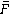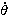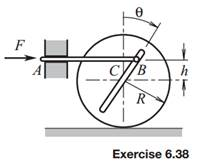### Create an Account

Home / Questions / The force in Exercise 638 is constant and frictional resistance between pin B and the gro...

# The force in Exercise 638 is constant and frictional resistance between pin B and the groove is negligible Initially θ 60° and 0 The distance h 06R Derive an expression for the angular

The forcein Exercise 6.38 is constant and frictional resistance between pin B and the groove is negligible. Initially, θ = −60° and= 0. The distance h = 0.6R. Derive an expression for the angular speed of the wheel when θ = 30°, based on the assumption that there is no slippage when the wheel rolls.

Exercise 6.38

Horizontal force F causes the actuating rod to move to the left at the constant speed v. This rod is connected to the wheel by pin B, which may slide through the groove. The mass of the wheel is m, the radius of gyration is κ, and µs and µk are the coefficients of static and kinetic friction, respectively, between the wheel and the ground. Friction between the pin and the groove is negligible, as is the mass of the rod. Consider the instant when θ = 30°. Derive expressions for the acceleration of center C, the angular acceleration of the gear, and the force F under the assumption that (a) the wheel rolls without slipping, (b) there is slippage between the wheel and the ground.Jun 16 2020 View more View LessSubscribe To Get Solution